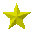Right!   correlation: most generally, the degree to which one phenomenon or random variable is associated with or can be predicted from another. In statistics, correlation usually refers to the degree to which a linear predictive relationship exists between random variables, as measured by a correlation coefficient (q.v.). Correlation may be positive (but never larger than 1), i.e., both variables increase or decrease together; negative or inverse (but never smaller than -1), i.e., one variable increases when the other decreases; or zero, i.e., a change in one variable does not affect the other.